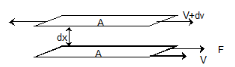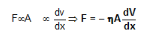Courses

# Doc: Liquid State Class 11 Notes | EduRev

## Class 11 : Doc: Liquid State Class 11 Notes | EduRev

The document Doc: Liquid State Class 11 Notes | EduRev is a part of the Class 11 Course Chemistry Class 11.
All you need of Class 11 at this link: Class 11

• The liquid molecules are relatively close together.
• The intermolecular forces of attraction in case of liquids are much larger than in gases.
• Unlike gases, liquids have a definite volume although no definite shape (similarity with gases).
• The molecules are in constant random motion.
• The average kinetic energy of molecules in a given sample is proportional to the absolute temperature.

Guldberg’s Rule

Normal boiling point of the liquid is nearly two – third of its critical temperature when both are expressed on the absolute scale. Tb = 2/3 Tc

Trouton’s Rule

The molar heat of vapourisation of a liquid expressed in Joules divided by normal b.p. of the liquid on the absolute scale is approximately equal to 88. ΔHv / Tb.p. = 88 JK–1 mol–1

Evaporation

Evaporation is the spontaneous change in which a liquid changes into vapours at the surface of liquid. Evaporation occurs at all temperatures. Evaporation increases with increase in surface area, increase in temperature and decrease in intermolecular attractive forces. In contrast to evaporation, boiling takes place at a definite temperature and it involves bubble formation below the surface. Evaporation produces cooling.

## Vapour Pressure

• Vapour pressure of a liquid at any temperature may be defined as the pressure exerted by the vapour present above the liquid in equilibrium with the liquid at that temperature. The magnitude of vapour pressure depends upon the nature of liquid and temperature.
• Non – polar or less polar liquids have fairly high vapour pressure due to weak forces of attraction (e.g. ether etc). Polar liquids (e.g. water, alcohols, etc.) have lower vapour pressure because of strong dipole – dipole interaction between their molecules.
• Vapour pressure of a liquid is constant at a given temperature. Further the vapour pressure of a liquid increases with increase in temperature. When the vapour pressure of the liquid is equal to the external pressure (normal pressure or 1 atm pressure) acting upon the surface of the liquid, the bubbles increase in size and escape freely; the temperature at which this happens is called the boiling point of the liquid. In case the external pressure is more than the atmospheric pressure, more heat will be required to make the vapour pressure equal to the external pressure and hence higher will be the boiling point. In case, the external pressure is low (as on the top of a mountain), the boiling point of the liquid decreases. This explains why a liquid boils at a lower temperature on the top of a mountain. (where pressure in low) than on the sea shore.
• Substances having high vapour pressure (e.g. petrol) evaporate more quickly than substances of low vapour pressure. (e.g. motor oil)

Surface Tension

• Surface Tension of a liquid is defined as the force acting at right angles to the surface along one centimeter length of the surface. It is represented by the greek letter gamma, γ
• Due to surface tension molecules tend to leave the surface, i.e. the surface of the liquid tends to contract to the smallest possible area for a given volume of the liquid.
• Further for a given volume of the liquid, sphere has the minimum surface area. This explains why the drops of a liquid are spherical.
• Thus it is apparent that in order to increase its surface area, force must be exerted to overcome the surface tension. In other words, work will have to be done to increase the surface area.
• Thus the surface tension of a liquid is defined as the work (energy) required to expand the surface of a liquid by unit area.
• Mathematically: Surface tension = Work done / Change in area
• Thus surface tension of a liquid may also be defined as the force in dynes necessary to rupture its surface along one centimeter length. In SI units it is defined as the force in newtons  required to rupture 1 meter length of the surface of a liquid.
• Thus the units of surface tension are dynescm-1 (or Newtons per metre, in SI system).
• Surface tension of a liquid is measured with the help of apparatus called stalagmometer.
• The surface tension of a liquid decreases with increase of temperature and becomes zero at its critical temperature (where the surface of separation between liquid and its vapour disappears).
• The decrease in surface tension with increase of temperature is due to the fact that with increase of temperature, the kinetic energy of the molecule (and hence the speed of molecules) increases and hence the intermolecular forces of attraction decreases.

Viscosity

• Definition: When a fluid flows through channel  the fluid layer in the bottom is in  contact with the channel remains at rest. The upper most fluid layer moves with maximum velocity. Thus there is a velocity gradient existing among different layers of fluid. The faster moving layer intends the slower layer in contact to accelerate and simultaneously the slower moving layer intends the faster moving layer to retard. This property of liquids is called viscosity. The force existing between the two consecutive layers of fluids due to their velocity gradient is called viscous force.Let us consider two layers of fluid each of surface area A. The viscous force (F) between them isWhere n is the coefficient of viscosity of the fluids.

• Coefficient of viscosity may be defined as the force per unit area required to maintain unit difference of velocity between two parallel layers in the liquid, one unit apart. In C.G.S. units, it is expressed in dynes per square centimeter. This unit is called a poise after the name of Poiseulle who pioneered the study of viscosity. Low values of viscosity are expressed in centipoise and millipoise. In S.I. units, viscosity is expressed in  or Pas (pascal second).   Thus, the coefficient of viscosity of a liquid is viscous force acting tangentially per unit area of liquid layer having unit velocity gradient in a direction perpendicular to the direction of flow of liquid.
• Unit of coefficient of viscosity is poise.
• Strokes Law:When a body falls through a viscous medium (liquid or gas), it drags the layer of the fluid immediately in contact with it. This produces a relative motion between the different layers of the fluid. As a result of this, the falling body experiences a viscous force. This force tends to retard the motion of the body.
• Stokes performed many experiments on the motion of small spherical bodies in different fluids. He concluded that the viscous forces F acting on a small sphere of radius r depends upon (I) coefficient of viscosity (h) of the fluid, (ii) velocity (v) of the spherical body and (iii) the radius r.
• Viscous force F is given by the following formula
• F= 6πηrv
• The viscosity of a liquid generally decreases with rise in temperature. With increase of temperature, the kinetic energy of the molecules of the liquid increases and hence the liquid starts flowing faster, i.e. the viscosity decreases. The decrease in viscosity is found to be about 3% per degree rise of temperature.
• Lubricating oils are graded according to their viscosity. A good quality or ‘all – weather’ lubricating is one whose viscosity and hence lubricating property does not change much with increase of temperature. Such oils are obtained by adding long chain coiling polymers to the oil. As temperature rises, the polymer particles tend to uncoil and thus increase the viscosity of oil thereby compensating for the decrease of viscosity of the oil with rise of temperature.
• The condition of high blood pressure and thus strain on heart may also be explained on the property of viscosity. In arteriosclerosis (hardening of arteries), arterial walls contract resulting in decrease of diameter of capillaries.
• Narrow capillaries offer resistance to the flow of blood due to viscosity with the result greater force is needed to make blood flow through capillaries. This results in a condition of high blood pressure and strain on the heart.
• The increased blood circulation required during fever is supplied by its temperature dependence properly (recall that rise of every degree centigrade temperature decreases viscosity of blood by about 3%). Thus lowering of viscosity results in a more rapid flow of blood without any extra strain on the heart.
• In case of asphyxia, concentration of CO in blood increases resulting in swelling of corpuscles which then increases viscosity of blood.
Offer running on EduRev: Apply code STAYHOME200 to get INR 200 off on our premium plan EduRev Infinity!

,

,

,

,

,

,

,

,

,

,

,

,

,

,

,

,

,

,

,

,

,

;